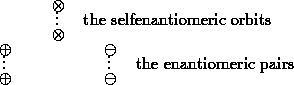Splitting Orbits

### Splitting Orbits

In the case when GX is a finite action, we can apply the sign map e to bar (G), the permutation group induced by G on X. Its kernel
bar (G)+:= { bar (g) Îbar (G) | e( bar (g)) =1 }
is either bar (G) itself or a subgroup of index 2, as is easy to see. Denoting its inverse image by
G+:= {g ÎG | e( bar (g))=1 },
we obtain a useful interpretation of the alternating sum of fixed point numbers:
Lemma: For any finite action GX such that G not = G+, the number of orbits of G on X which split over G+ (i.e. which decompose into more than one -- and hence into two -- G+-orbits) is equal to
(1)/( | G | ) åg ÎG e( bar (g)) | Xg | =(1)/( | bar (G) | ) å bar (g) Îbar (G) e( bar (g)) | X bar (g) | .
Proof: As G not =G+, and hence | G+ | = | G | /2, we have
(1)/( | G | ) åg ÎG e( bar (g)) | Xg | =(2)/( | G | ) åg ÎG+ | Xg | - (1)/( | G | ) åg ÎG | Xg |
=(1)/( | G+ | ) åg ÎG+ | Xg | - (1)/( | G | ) åg ÎG | Xg | = | G+ \\X | - | G \\X | .
Each orbit of G on X is either a G+-orbit or it splits into two orbits of G+, since | G+ | = | G | /2. Hence | G+ \\X | - | G \\X | is the number of orbits which split over G+. Finally the stated identity is obtained by an application of the homomorphism theorem.
Corollary: In the case when G not = G+, the number of G-orbits on X which do not split over G+ is equal to
(1)/( | G | ) åg ÎG(1- e( bar (g))) | Xg | .
Note what this means. If G acts on a finite set X in such a way that G not =G+, then we can group the orbits of G on X into a set of orbits which are also G+-orbits. In the figure we denote these orbits by the symbol Ä. The other G-orbits split into two G+-orbits, we indicate one of them by Å, the other one by OMINUS , and call the pair { Å, OMINUS } an enantiomeric pair of G-orbits. Hence the Lemma above gives us the number of enantiomeric pairs of orbits, while corollary yields the number of selfenantiomeric orbits of G on X. The elements x ÎX belonging to selfenantiomeric orbits are called achiral objects, while the others are called chiral. These notions of enantiomerism and chirality are taken from chemistry, where G is usually the symmetry group of the molecule while G+ is its subgroup consisting of the proper rotations. We call GX a chiral action if and only if G not = G+.

Enantiomeric pairs and selfenantiomeric orbitsUsing this notation we can now rephrase aboves lemma and corollary in the following way:

Corollary: If GX is a finite chiral action, then the number of selfenantiomeric orbits of G on X is equal to
(1)/( | G | ) åg ÎG(1- e( bar (g))) | Xg | = 2 | G \\X | - | G+ \\X | ,
while the number of enantiomeric pairs of orbits is
(1)/( | G | ) åg ÎG e( bar (g)) | Xg | = | G+ \\X | - | G \\X | .
The sign of a cyclic permutation is easy to obtain from the equation and the homomorphism property of the sign, described in the corollary:
(i1 ...ir) ÎAn iff r is odd.
But in fact we need not check the lengths of the cyclic factors of p since an easy calculation shows (exercise) that, in terms of the number c( p) of cyclic factors of p, we have
e( p)=(-1)n-c( p), if pÎSn .

harald.fripertinger@kfunigraz.ac.at,
last changed: August 28, 2001Splitting Orbits